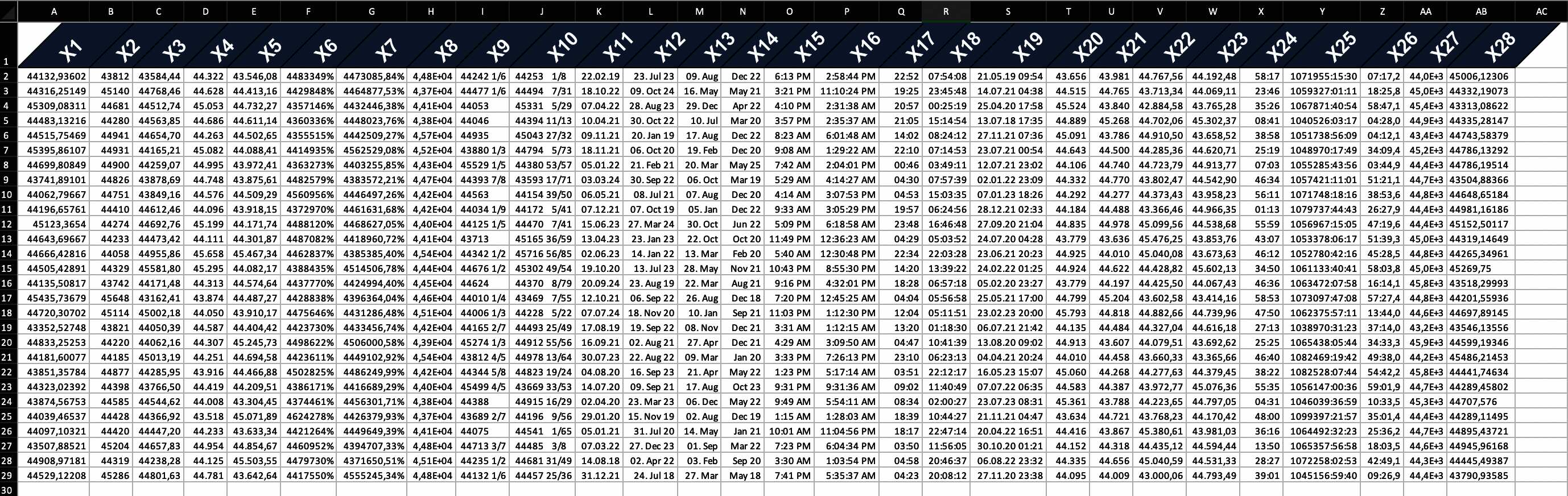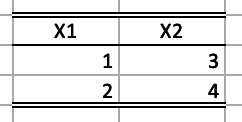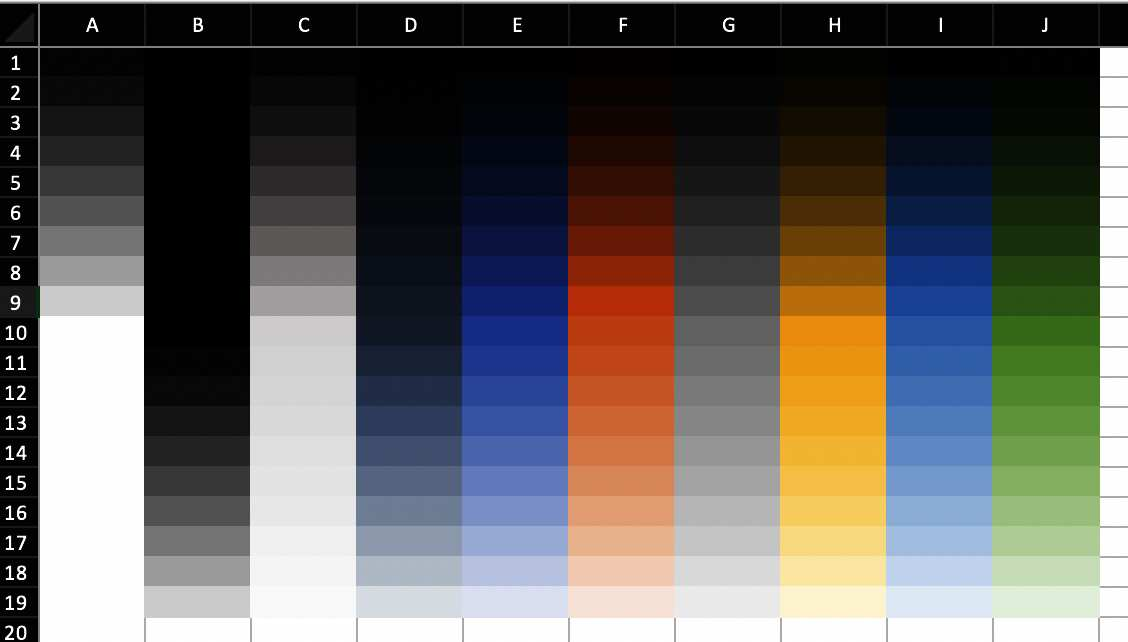# openxlsx2 style manual

Welcome to the styling manual for openxlsx2. In this manual you will learn how to use openxlsx2 to style your worksheets. data from xlsx-files to R as well as how to export data from R to xlsx, and how to import and modify these openxml workbooks in R.

# Styling showcase

## Colors, text rotation and number formats

Below we show you two ways how to create styled tables with openxlsx2 one using the high level functions to style worksheet areas and one using the bare metal approach of creating the identical table. We show both ways to create styles in openxlsx2 to show how you could build on our functions or create your very own functions.The example below, with increased column width.

### the quick way: using high level functions

# add some dummy data
set.seed(123)
mat <- matrix(rnorm(28 * 28, mean = 44444, sd = 555), ncol = 28)
colnames(mat) <- make.names(seq_len(ncol(mat)))
border_col <- wb_color(theme = 1)
border_sty <- "thin"

# prepare workbook with data and formated first row
wb <- wb_workbook() %>%
top_color = border_col, top_border = border_sty,
bottom_color = border_col, bottom_border = border_sty,
left_color = border_col, left_border = border_sty,
right_color = border_col, right_border = border_sty,
inner_hcolor = border_col, inner_hgrid = border_sty
) %>%
wb_add_fill(dims = "A1:AB1", color = wb_color(hex = "FF334E6F")) %>%
wb_add_font(dims = "A1:AB1", name = "Arial", bold = TRUE, color = wb_color(hex = "FFFFFFFF"), size = 20) %>%
wb_add_cell_style(dims = "A1:AB1", horizontal = "center", textRotation = 45)

# create various number formats
x <- c(
0, 1, 2, 3, 4, 9, 10, 11, 12, 13, 14, 15, 16, 17, 18, 19, 20, 21, 22,
37, 38, 39, 40, 45, 46, 47, 48, 49
)

# apply the styles
for (i in seq_along(x)) {
cell <- sprintf("%s2:%s29", int2col(i), int2col(i))
wb <- wb %>% wb_add_numfmt(dims = cell, numfmt = x[i])
}

# wb$open() ### the long way: using bare metal functions # create workbook wb <- wb_workbook() %>% wb_add_worksheet("test") # add some dummy data to the worksheet set.seed(123) mat <- matrix(rnorm(28 * 28, mean = 44444, sd = 555), ncol = 28) colnames(mat) <- make.names(seq_len(ncol(mat))) wb$add_data(x = mat, colNames = TRUE)

# create a border style and assign it to the workbook
black <- wb_color(hex = "FF000000")
new_border <- create_border(
bottom = "thin", bottom_color = black,
top = "thin", top_color = black,
left = "thin", left_color = black,
right = "thin", right_color = black
)
wb$styles_mgr$add(new_border, "new_border")

# create a fill style and assign it to the workbook
new_fill <- create_fill(patternType = "solid", fgColor = wb_color(hex = "FF334E6F"))
wb$styles_mgr$add(new_fill, "new_fill")

# create a font style and assign it to the workbook
new_font <- create_font(sz = 20, name = "Arial", b = TRUE, color = wb_color(hex = "FFFFFFFF"))
wb$styles_mgr$add(new_font, "new_font")

# create a new cell style, that uses the fill, the font and the border style
new_cellxfs <- create_cell_style(
numFmtId = 0,
horizontal = "center",
textRotation = 45,
fillId = wb$styles_mgr$get_fill_id("new_fill"),
fontId = wb$styles_mgr$get_font_id("new_font"),
borderId = wb$styles_mgr$get_border_id("new_border")
)
# assign this style to the workbook
wb$styles_mgr$add(new_cellxfs, "new_styles")

# assign the new cell style to the header row of our data set
cell <- sprintf("A1:%s1", int2col(nrow(mat)))
wb <- wb %>% wb_set_cell_style(
dims = cell,
style = wb$styles_mgr$get_xf_id("new_styles")
)

## style the cells with some builtin format codes (no new numFmt entry is needed)
x <- c(
1, 2, 3, 4, 9, 10, 11, 12, 13, 14, 15, 16, 17, 18, 19, 20, 21, 22,
37, 38, 39, 40, 45, 46, 47, 48, 49
)

# create styles
new_cellxfs <- create_cell_style(numFmtId = x, horizontal = "center")

# assign the styles to the workbook
for (i in seq_along(x)) {
wb$styles_mgr$add(new_cellxfs[i], paste0("new_style", i))
}

# new styles are 1:28
new_styles <- wb$styles_mgr$get_xf()
for (i in as.integer(new_styles$id[new_styles$name %in% paste0("new_style", seq_along(x))])) {
cell <- sprintf("%s2:%s29", int2col(i), int2col(i))
wb <- wb %>% wb_set_cell_style(dims = cell, style = i)
}

# assign a custom tabColor
wb$worksheets[]$sheetPr <- xml_node_create(
"sheetPr",
xml_children = xml_node_create(
"tabColor",
xml_attributes = wb_color(hex = "FF00FF00")
)
)

# # look at the beauty you've created
# wb_open(wb)

# Working with number formats

Per default openxlsx2 will pick up number formats for selected R classes.

## numfmts

## Create Workbook object and add worksheets
wb <- wb_workbook()
wb$add_worksheet("S1") wb$add_worksheet("S2")

df <- data.frame(
"Date" = Sys.Date() - 0:19,
"T" = TRUE, "F" = FALSE,
"Time" = Sys.time() - 0:19 * 60 * 60,
"Cash" = paste("$", 1:20), "Cash2" = 31:50, "hLink" = "https://CRAN.R-project.org/", "Percentage" = seq(0, 1, length.out = 20), "TinyNumbers" = runif(20) / 1E9, stringsAsFactors = FALSE ) ## openxlsx will apply default Excel styling for these classes class(df$Cash) <- c(class(df$Cash), "currency") class(df$Cash2) <- c(class(df$Cash2), "accounting") class(df$hLink) <- "hyperlink"
class(df$Percentage) <- c(class(df$Percentage), "percentage")
class(df$TinyNumbers) <- c(class(df$TinyNumbers), "scientific")

wb$add_data("S1", x = df, startRow = 4, rowNames = FALSE) wb$add_data_table("S2", x = df, startRow = 4, rowNames = FALSE)

## numfmts2

In addition, you can set the style to be picked up using openxlsx2 options.

wb <- wb_workbook()

options("openxlsx2.dateFormat" = "yyyy")
options("openxlsx2.datetimeFormat" = "yyyy-mm-dd")
options("openxlsx2.numFmt" = "€ #.0")

df <- data.frame(
"Date" = Sys.Date() - 0:19,
"T" = TRUE, "F" = FALSE,
"Time" = Sys.time() - 0:19 * 60 * 60,
"Cash" = paste("$", 1:20), "Cash2" = 31:50, "hLink" = "https://CRAN.R-project.org/", "Percentage" = seq(0, 1, length.out = 20), "TinyNumbers" = runif(20) / 1E9, stringsAsFactors = FALSE, "numeric" = 1 ) ## openxlsx will apply default Excel styling for these classes class(df$Cash) <- c(class(df$Cash), "currency") class(df$Cash2) <- c(class(df$Cash2), "accounting") class(df$hLink) <- "hyperlink"
class(df$Percentage) <- c(class(df$Percentage), "percentage")
class(df$TinyNumbers) <- c(class(df$TinyNumbers), "scientific")

wb$add_data("test", df) # Modifying the column widths ## wb_set_col_widths wb <- wb_workbook() %>% wb_add_worksheet() %>% wb_add_data(x = mtcars, rowNames = TRUE) cols <- 1:12 wb <- wb %>% wb_set_col_widths(cols = cols, widths = "auto") # Adding borders ## add borders wb <- wb_workbook() # full inner grid wb$add_worksheet("S1", gridLines = FALSE)$add_data(x = mtcars) wb$add_border(
dims = "A2:K33",
inner_hgrid = "thin", inner_hcolor = wb_color(hex = "FF808080"),
inner_vgrid = "thin", inner_vcolor = wb_color(hex = "FF808080")
)
# only horizontal grid
wb$add_worksheet("S2", gridLines = FALSE)$add_data(x = mtcars)
wb$add_border(dims = "A2:K33", inner_hgrid = "thin", inner_hcolor = wb_color(hex = "FF808080")) # only vertical grid wb$add_worksheet("S3", gridLines = FALSE)$add_data(x = mtcars) wb$add_border(dims = "A2:K33", inner_vgrid = "thin", inner_vcolor = wb_color(hex = "FF808080"))
# no inner grid
wb$add_worksheet("S4", gridLines = FALSE)$add_data(x = mtcars)
wb$add_border("S4", dims = "A2:K33") ## styled table Below we show you two ways how to create styled tables with openxlsx2 one using the high level functions to style worksheet areas and one using the bare metal approach of creating the identical table.### the quick way: using high level functions # add some dummy data to the worksheet mat <- matrix(1:4, ncol = 2, nrow = 2) colnames(mat) <- make.names(seq_len(ncol(mat))) wb <- wb_workbook() %>% wb_add_worksheet("test") %>% wb_add_data(x = mat, colNames = TRUE, startCol = 2, startRow = 2) %>% # center first row wb_add_cell_style(dims = "B2:C2", horizontal = "center") %>% # add border for first row wb_add_border( dims = "B2:C2", bottom_color = wb_color(theme = 1), bottom_border = "thin", top_color = wb_color(theme = 1), top_border = "double", left_border = NULL, right_border = NULL ) %>% # add border for last row wb_add_border( dims = "B4:C4", bottom_color = wb_color(theme = 1), bottom_border = "double", top_border = NULL, left_border = NULL, right_border = NULL ) ### the long way: creating everything from the bone # add some dummy data to the worksheet mat <- matrix(1:4, ncol = 2, nrow = 2) colnames(mat) <- make.names(seq_len(ncol(mat))) wb <- wb_workbook() %>% wb_add_worksheet("test") %>% wb_add_data(x = mat, startCol = 2, startRow = 2) # create a border style and assign it to the workbook black <- wb_color(hex = "FF000000") top_border <- create_border( top = "double", top_color = black, bottom = "thin", bottom_color = black ) bottom_border <- create_border(bottom = "double", bottom_color = black) wb$styles_mgr$add(top_border, "top_border") wb$styles_mgr$add(bottom_border, "bottom_border") # create a new cell style, that uses the fill, the font and the border style top_cellxfs <- create_cell_style( numFmtId = 0, horizontal = "center", borderId = wb$styles_mgr$get_border_id("top_border") ) bottom_cellxfs <- create_cell_style( numFmtId = 0, borderId = wb$styles_mgr$get_border_id("bottom_border") ) # assign this style to the workbook wb$styles_mgr$add(top_cellxfs, "top_styles") wb$styles_mgr$add(bottom_cellxfs, "bottom_styles") # assign the new cell style to the header row of our data set cell <- "B2:C2" wb <- wb %>% wb_set_cell_style(dims = cell, style = wb$styles_mgr$get_xf_id("top_styles")) cell <- "B4:C4" wb <- wb %>% wb_set_cell_style(dims = cell, style = wb$styles_mgr$get_xf_id("bottom_styles")) # Use workbook colors and modify them The loop below will apply the tint attribute to the fill colorTint variations of the theme colors. wb <- wb_workbook() %>% wb_add_worksheet("S1") tints <- seq(-0.9, 0.9, by = 0.1) for (i in 0:9) { for (tnt in tints) { col <- paste0(int2col(i + 1), which(tints %in% tnt)) if (tnt == 0) { wb <- wb %>% wb_add_fill(dims = col, color = wb_color(theme = i)) } else { wb <- wb %>% wb_add_fill(dims = col, color = wb_color(theme = i, tint = tnt)) } } } # Copy cell styles It is possible to copy the styles of several cells at once. In the following example, the styles of some cells from a formatted workbook are applied to a previously empty cell range. Be careful though, wb_get_cell_style() returns only some styles, so you have to make sure that the copy-from and copy-to dimensions match in a meaningful way. wb <- wb_load(system.file("extdata", "oxlsx2_sheet.xlsx", package = "openxlsx2")) %>% wb_set_cell_style(1, "A30:G35", wb_get_cell_style(., 1, "A10:G15")) # wb_open(wb) # Style strings Using fmt_txt() is possible to style strings independently of the cell containing the string. txt <- paste( fmt_txt("Embracing the full potential of "), fmt_txt("openxlsx2", bold = TRUE, size = 16), fmt_txt(" with "), fmt_txt("fmt_txt()", font = "Courier"), fmt_txt(" !") ) wb <- wb_workbook()$add_worksheet()$add_data(x = txt) As shown above it is possible to combine multiple styles together into a longer string. It is even possible to use fmt_txt() as na.strings: df <- mtcars df[df < 4] <- NA na_red <- fmt_txt("N/A", color = wb_color("red"), italic = TRUE, bold = TRUE) wb <- wb_workbook()$add_worksheet()$add_data(x = df, na.strings = na_red) # Create custom table styles With create_tablestyle() it is possible to create your own table styles. This function uses create_dxfs_style() (just like your spreadsheet software does). Therefore, it is not quite as user-friendly. The following example shows how the function creates a red table style. The various dxfs styles must be created and assigned to the workbook (similar styles are used in conditional formatting). In create_tablestyle() these styles are assigned to the table style elements. Once the table style is created, it must also be assigned to the workbook. After that you can use it in the workbook like any other table style. # a red table style dx0 <- create_dxfs_style( border = TRUE, left_color = wb_color("red"), right_color = NULL, right_style = NULL, top_color = NULL, top_style = NULL, bottom_color = NULL, bottom_style = NULL ) dx1 <- create_dxfs_style( border = TRUE, left_color = wb_color("red"), right_color = NULL, right_style = NULL, top_color = NULL, top_style = NULL, bottom_color = NULL, bottom_style = NULL ) dx2 <- create_dxfs_style( border = TRUE, top_color = wb_color("red"), left_color = NULL, left_style = NULL, right_color = NULL, right_style = NULL, bottom_color = NULL, bottom_style = NULL ) dx3 <- create_dxfs_style( border = TRUE, top_color = wb_color("red"), left_color = NULL, left_style = NULL, right_color = NULL, right_style = NULL, bottom_color = NULL, bottom_style = NULL ) dx4 <- create_dxfs_style( text_bold = TRUE ) dx5 <- create_dxfs_style( text_bold = TRUE ) dx6 <- create_dxfs_style( font_color = wb_color("red"), text_bold = TRUE, border = TRUE, top_style = "double", left_color = NULL, left_style = NULL, right_color = NULL, right_style = NULL, bottom_color = NULL, bottom_style = NULL ) dx7 <- create_dxfs_style( font_color = wb_color("white"), text_bold = TRUE, bgFill = wb_color("red"), fgColor = wb_color("red") ) dx8 <- create_dxfs_style( border = TRUE, left_color = wb_color("red"), top_color = wb_color("red"), right_color = wb_color("red"), bottom_color = wb_color("red") ) wb <- wb_workbook() %>% wb_add_worksheet(gridLines = FALSE) wb$add_style(dx0)
wb$add_style(dx1) wb$add_style(dx2)
wb$add_style(dx3) wb$add_style(dx4)
wb$add_style(dx5) wb$add_style(dx6)
wb$add_style(dx7) wb$add_style(dx8)

# finally create the table
xml <- create_tablestyle(
name               = "red_table",
wholeTable         = wb$styles_mgr$get_dxf_id("dx8"),
headerRow          = wb$styles_mgr$get_dxf_id("dx7"),
totalRow           = wb$styles_mgr$get_dxf_id("dx6"),
firstColumn        = wb$styles_mgr$get_dxf_id("dx5"),
lastColumn         = wb$styles_mgr$get_dxf_id("dx4"),
firstRowStripe     = wb$styles_mgr$get_dxf_id("dx3"),
secondRowStripe    = wb$styles_mgr$get_dxf_id("dx2"),
firstColumnStripe  = wb$styles_mgr$get_dxf_id("dx1"),
secondColumnStripe = wb$styles_mgr$get_dxf_id("dx0")
)

wb_add_data_table(x = mtcars, tableStyle = "red_table")